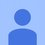# Help: Applying Quantum Gates in Order

In Quantum Computing --> Information --> Quiz 3: Gates Galore --> Problem 10, three quantum gates are applied, in the following order: "first X, then Z, and then H".

I tried to solve this by turning HZX into a single operation using matrix multiplication. However, I seem to have ordered my multiplication incorrectly. I thought that the order would be (X times Z) times H, which works out to the correct answer of $\frac{1}{\sqrt2}\begin{bmatrix} -1 & 1\\ 1 & 1 \end{bmatrix}$

However, the order shown in the explanation (which gives the same answer) is H times (Z times X).

Which is correct, and why?

P.S. Quantum Computing does not show up as a Topic when making a post, so I labelled this "Computer Science".Note by Adrian Self
2 years, 2 months ago

This discussion board is a place to discuss our Daily Challenges and the math and science related to those challenges. Explanations are more than just a solution — they should explain the steps and thinking strategies that you used to obtain the solution. Comments should further the discussion of math and science.

When posting on Brilliant:

• Use the emojis to react to an explanation, whether you're congratulating a job well done , or just really confused .
• Ask specific questions about the challenge or the steps in somebody's explanation. Well-posed questions can add a lot to the discussion, but posting "I don't understand!" doesn't help anyone.
• Try to contribute something new to the discussion, whether it is an extension, generalization or other idea related to the challenge.
• Stay on topic — we're all here to learn more about math and science, not to hear about your favorite get-rich-quick scheme or current world events.

MarkdownAppears as
*italics* or _italics_ italics
**bold** or __bold__ bold
- bulleted- list
• bulleted
• list
1. numbered2. list
1. numbered
2. list
Note: you must add a full line of space before and after lists for them to show up correctly
paragraph 1paragraph 2

paragraph 1

paragraph 2

[example link](https://brilliant.org)example link
> This is a quote
This is a quote
    # I indented these lines
# 4 spaces, and now they show
# up as a code block.

print "hello world"
# I indented these lines
# 4 spaces, and now they show
# up as a code block.

print "hello world"
MathAppears as
Remember to wrap math in $$ ... $$ or $ ... $ to ensure proper formatting.
2 \times 3 $2 \times 3$
2^{34} $2^{34}$
a_{i-1} $a_{i-1}$
\frac{2}{3} $\frac{2}{3}$
\sqrt{2} $\sqrt{2}$
\sum_{i=1}^3 $\sum_{i=1}^3$
\sin \theta $\sin \theta$
\boxed{123} $\boxed{123}$

## Comments

Sort by:

Top Newest

As I understand, we usually understand $\ket{0}$ to be the vector $\begin{bmatrix}0\\ 1\end{bmatrix}$. So, in order to apply the function (or linear transformation) $X$ on $\ket{0}$, one says $X\ket{0}$. Seen this way, we need to figure out $HZX\ket{0}$

- 2 years, 1 month ago

Log in to reply

Interestingly, the next problem is, "Would the resulting state always be the same if we had applied the gates in a different order?"

- 2 years, 2 months ago

Log in to reply

In general, matrix multiplication is non-commutative (meaning that the order is important). Try out the order shown in the problem and see if it works out to your answer. If it doesn't, there is probably a typo in the quiz.

- 2 years, 2 months ago

Log in to reply

×

Problem Loading...

Note Loading...

Set Loading...